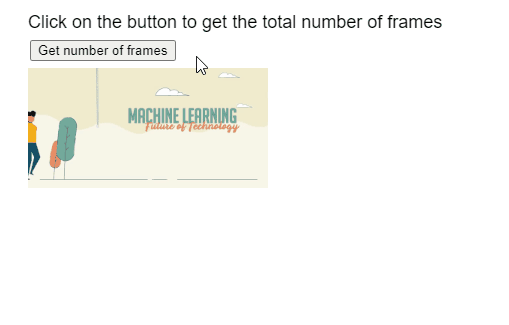GeeksforGeeks App
Open AppBrowser
Continue

# p5.js Image numFrames() Method

The numFrames() method of p5.Image in p5.js library is used to return the total number of frames of the GIF animation.

Syntax:

``` numFrames()
```

Parameters: This function accept does not accept any parameter.

Return Value: This method returns a number that represents the total number of frames of the GIF animation.

The following libraries are included in the “head” section of the HTML page while implementing the following examples.

<script src=”p5.Image.js”></script>
<script src=”p5.min.js”></script>

Example: The example below illustrates the numFrames() method in p5.js

## Javascript

 `function` `preload() {``    ``example_gif =``      ``loadImage(``"sample-gif.gif"``);``}`` ` `function` `setup() {``    ``createCanvas(500, 300);``    ``textSize(18);`` ` `    ``text(``"Click on the button to get "` `+``         ``"the total number of frames"``,``         ``20, 20);`` ` `    ``frameBtn =``      ``createButton(``"Get number of frames"``);``    ``frameBtn.position(30, 40);``    ``frameBtn.mousePressed(getTotalFrames);``}`` ` `function` `draw() {`` ` `  ``// Draw the GIF on screen``  ``image(example_gif, 20, 60, 240, 120);``}`` ` `function` `getTotalFrames()``{``  ``// Get the total number of frames``  ``let numberOfFrames =``      ``example_gif.numFrames();`` ` `  ``text(``"Number of frames in the GIF is: "``       ``+ numberOfFrames, 20, 200);``}`

Output:Online editor: https://editor.p5js.org/
Environment Setup: https://www.geeksforgeeks.org/p5-js-soundfile-object-installation-and-methods/
Reference: https://p5js.org/reference/#/p5.Image/numFrames

My Personal Notes arrow_drop_up
Related Tutorials